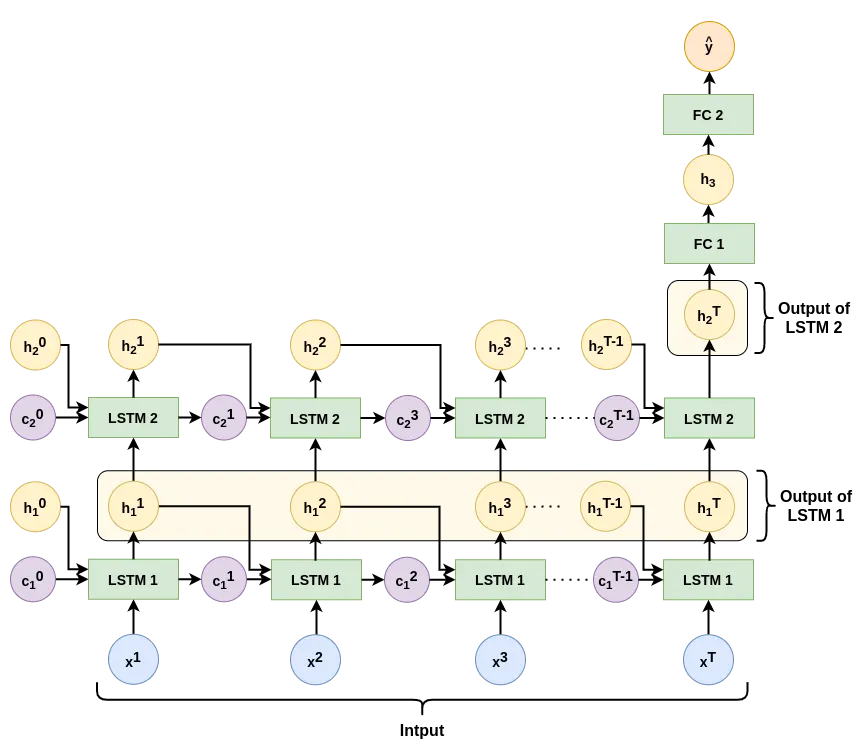# Difference between sequence length and hidden size in LSTM

It does not come clear to me how the seq_length is not the exact same as the hidden_size in LSTMs.

For example, in the next image, the seq_length is T since there are $$x_{1}, x_{2}, ..., x_{T}$$ time steps and there are is one LSTM cell (green LSTM blocks) per input $$x_{i}$$. So in this image seq_length == hidden_sizeIn addition, every LSTM cell requires 3 inputs:

1. $$x_{t}$$ the input value at time t.
2. $$h_{t-1}$$ the hidden state value from the previous time step.
3. $$c_{t-1}$$ the cell state value from the previous time step.

This can be seen in the following image:How can the hidden_size be lower or higher than the seq_length? In any of both cases the LSTM cells would not have the $$x_{i}$$ input which is required.